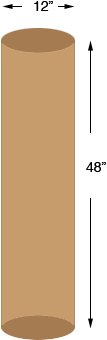Question: I have 15 tubes. Each tube measures 12in.x 48in. I have to fill each one with concrete. How do I figure how many cubic yards of concrete I will need? Thanks Paul Paul, I assume that the tubes are 12 in in diameter and 48" long.The volume of a cylinder isr2 h where r is the radius and h is the height. You have the dimensions in inches so first you need to convert them to yards. There are 36 inches in a yard so r = 12/2 = 6 inches = 6/36 yards and h = 48/36  yards Thus the volume in cubic yards is( 6/36 )2( 48/36 ) = 0.116 But you have 15 such cylinders so you need 150.116 = 1.75 cubic yards of concrete. Penny